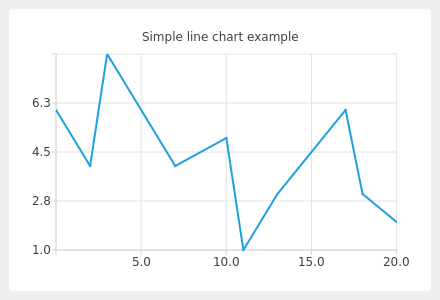# Line Chart Example¶

The example shows how to create a simple line chart.```"""PySide6 port of the linechart example from Qt v5.x"""

import sys
from PySide6.QtCore import QPointF
from PySide6.QtGui import QPainter
from PySide6.QtWidgets import QMainWindow, QApplication
from PySide6.QtCharts import QChart, QChartView, QLineSeries

class TestChart(QMainWindow):
def __init__(self):
super().__init__()

self.series = QLineSeries()
self.series.append(0, 6)
self.series.append(2, 4)
self.series.append(3, 8)
self.series.append(7, 4)
self.series.append(10, 5)
self.series.append(QPointF(11, 1))
self.series.append(QPointF(13, 3))
self.series.append(QPointF(17, 6))
self.series.append(QPointF(18, 3))
self.series.append(QPointF(20, 2))

self.chart = QChart()
self.chart.legend().hide()
self.chart.createDefaultAxes()
self.chart.setTitle("Simple line chart example")

self._chart_view = QChartView(self.chart)
self._chart_view.setRenderHint(QPainter.Antialiasing)

self.setCentralWidget(self._chart_view)

if __name__ == "__main__":
app = QApplication(sys.argv)

window = TestChart()
window.show()
window.resize(440, 300)
sys.exit(app.exec())
```